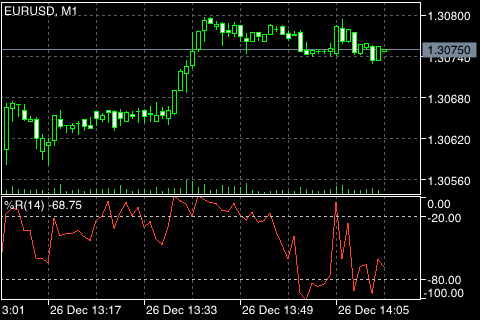威廉姆斯百分比范围计算

%R = -(MAX (HIGH (i - n)) - CLOSE (i)) / (MAX (HIGH (i - n)) - MIN (LOW (i - n))) * 100

CLOSE (i) ― 本期收盘价;
MAX (HIGH (i - n)) ― 之前 n 周期最高价的最大值;
MIN (LOW (i - n)) ― 之前 n 周期最低价的最小值。